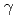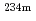Authors developed the new analysis technique (hereinafter referred to as the equivalent model) which calculates the amount of uranium by correcting the influence of uneven distribution of the uranium. Tworays different in the shelter effect are used in the equivalent model. Therays (766 keV, 1001 keV ) released fromPa are used for uranium quantitative determination. The quantity error is decided by theray with the small calculation rate. The way to get the high calculation rate is considered to reduce the quantity error. Manyrays are scattered by the Compton effect in radioactive waste, and scattered photons occur. We applied the scattered photon with the big count rate to equivalent model. It was effective to apply the count rate of the scattered photon by the Compton effect to equivalent model.